2022: SklogWiki celebrates 15 years on-line

# COMPASS force field

(diff) ← Older revision | Latest revision (diff) | Newer revision → (diff)

COMPASS (Condensed-phase Optimized Molecular Potentials for Atomistic Simulation Studies)      is a member of the consistent family of force fields (CFF91, PCFF, CFF and COMPASS), which are closely related second-generation force fields. They were parameterized against a wide range of experimental observables for organic compounds containing H, C, N, O, S, P, halogen atoms and ions, alkali metal cations, and several biochemically important divalent metal cations. PCFF is based on CFF91, extended so as to have a broad coverage of organic polymers, (inorganic) metals, and zeolites. COMPASS is the first force field that has been parameterized and validated using condensed phase properties in addition to various and empirical data for molecules in isolation. Consequently, this forcefield enables accurate and simultaneous prediction of structural, conformational, vibrational, and thermo-physical properties for a broad range of molecules in isolation and in condensed phases.

## Functional form

The COMPASS force field (Eq. 1 in Ref. 1) consists of terms for bonds (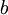$b$), angles (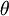$\theta$), dihedrals (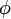$\phi$), out-of-plane angles (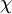$\chi$) as well as cross-terms, and two non-bonded functions, a Coulombic function for electrostatic interactions and a 9-6 Lennard-Jones potential for van der Waals interactions.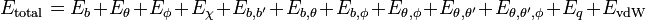$E_{\mathrm{total}} = E_b + E_{\theta} + E_{\phi} + E_{\chi} + E_{b,b'} + E_{b,\theta} + E_{b,\phi} + E_{\theta,\phi} + E_{\theta,\theta'} + E_{\theta,\theta',\phi} + E_{q} + E_{\mathrm{vdW}}$

where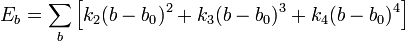$E_b = \sum_b \left[ k_2 (b-b_0)^2 + k_3 (b-b_0)^3 + k_4 (b-b_0)^4 \right]$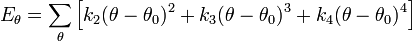$E_{\theta} = \sum_{\theta} \left[ k_2 (\theta-\theta_0)^2 + k_3 (\theta-\theta_0)^3 + k_4 (\theta-\theta_0)^4 \right]$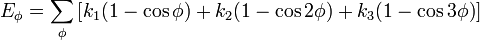$E_{\phi} = \sum_{\phi} \left[ k_1(1-\cos \phi) + k_2(1-\cos 2\phi) + k_3(1-\cos 3\phi) \right]$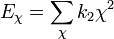$E_{\chi} = \sum_{\chi} k_2 \chi^2$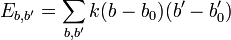$E_{b,b'} = \sum_{b,b'} k(b-b_0)(b'-b_0')$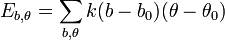$E_{b,\theta} = \sum_{b,\theta} k(b-b_0)(\theta-\theta_0)$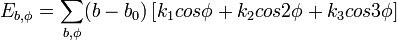$E_{b,\phi} = \sum_{b,\phi} (b-b_0) \left[k_1 cos \phi + k_2 cos 2\phi + k_3 cos 3\phi\right]$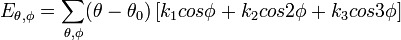$E_{\theta,\phi} = \sum_{\theta,\phi} (\theta-\theta_0) \left[k_1 cos \phi + k_2 cos 2\phi + k_3 cos 3\phi\right]$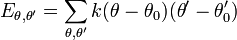$E_{\theta,\theta'} = \sum_{\theta,\theta'} k(\theta-\theta_0)(\theta'-\theta_0')$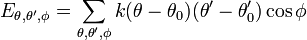$E_{\theta,\theta',\phi} = \sum_{\theta,\theta',\phi} k(\theta-\theta_0)(\theta'-\theta_0')\cos \phi$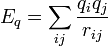$E_{q}=\sum_{ij} \frac{q_i q_j}{r_{ij}}$

and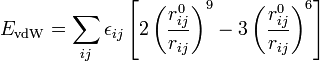$E_{\mathrm{vdW}}= \sum_{ij} \epsilon_{ij} \left[ 2 \left(\frac{r_{ij}^0}{r_{ij}} \right)^9 -3 \left(\frac{r_{ij}^0}{r_{ij}} \right)^6\right]$

with the Waldman-Hagler combining rules  (Note, in Ref. 1 Eq. 3 seems to have a typographical error):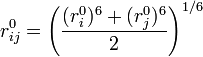$r_{ij}^0 = \left( \frac{ (r_i^0)^6 + (r_j^0)^6 }{2} \right)^{1/6}$

and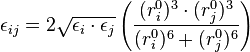$\epsilon_{ij} = 2 \sqrt{\epsilon_i \cdot \epsilon_j} \left( \frac{ (r_i^0)^3 \cdot (r_j^0)^3 }{ (r_i^0)^6 + (r_j^0)^6 } \right)$